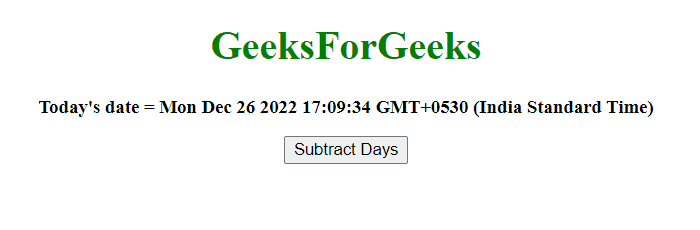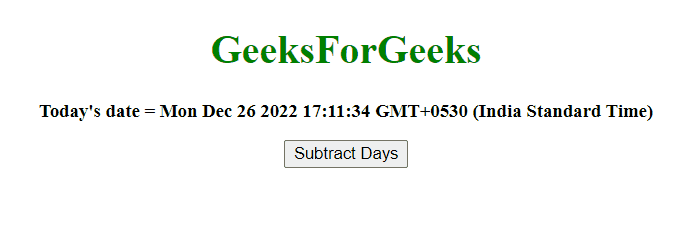GeeksforGeeks App
Open AppBrowser
Continue

# JavaScript Subtract days from Date object

Given a date and the task is to subtract days from the date. To subtract days from date in JavaScript, some methods are used which are described below:

JavaScript getDate() Method: This method returns the day of the month (from 1 to 31) for the defined date.

Syntax:

`Date.getDate()`

Parameters: This method does not accept any parameters.

Return value: It returns a number from 1 to 31, representing the day of the month.

JavaScript setDate() Method: This method sets the day of month to date object.

Syntax:

`Date.setDate(day)`

Parameters:

• day: It is required parameter. It specifies the integer representing the day of the month. Values expected values are 1-31, but other values are also allowed.
• 0 will result in the last day of the previous month.
• -1 will result in the day before the last day of the previous month.
• If the month has 31 days, 32 will result in the first day of the next month.
• If the month has 30 days, 32 will result in the second day of the next month.

Return value: This method return the number of milliseconds between the date object and midnight of January 1, 1970

JavaScript getTime() Method: This method returns the number of milliseconds between midnight of January 1, 1970, and the specified date.

Syntax:

`Date.getTime()`

Parameters: This method does not accept any parameters.

Return value: It returns a number, representing the number of milliseconds since midnight on January 1, 1970.

JavaScript setTime() Method: This method sets the date and time by adding/subtracting a defined number of milliseconds to/from midnight January 1, 1970.

Syntax:

`Date.setTime(millisec)`

Parameters:

• millisec: It is required parameter. It specifies the number of milliseconds to be added/subtracted, midnight January 1, 1970.

Return value: It represents the number of milliseconds between the date object and midnight January 1, 1970

Example 1: This example subtract 4 days from the var today by using setTime() and getTime() method.

## HTML

 `<``body` `style` `= ``"text-align:center;"``>``     ` `        ``<``h1` `style` `= ``"color:green;"` `>``            ``GeeksForGeeks``        ````         ` `        ``<``p` `id` `= ``"GFG_UP"` `style` `= ``"font-size: 15px; font-weight: bold;"``>``        ````         ` `        ``<``button` `onclick` `= ``"gfg_Run()"``>``            ``subtractDays``        ````         ` `        ``<``p` `id` `= ``"GFG_DOWN"` `style` `=``            ``"color:green; font-size: 20px; font-weight: bold;"``>``        ````         ` `        ``<``script``>``            ``var el_up = document.getElementById("GFG_UP");``            ``var el_down = document.getElementById("GFG_DOWN");``            ``var today = new Date();``            ``el_up.innerHTML = "Today's date = " + today;``             ` `            ``Date.prototype.subtractDays = function(d) {``                ``this.setTime(this.getTime() - (d*24*60*60*1000));``                ``return this;``            ``}``             ` `            ``function gfg_Run() {``                ``var a = new Date();``                ``a.subtractDays(4);``                ``el_down.innerHTML = a;``            ``}        ``        `````

Output:Subtract days from Date object

Example 2: This example subtract 365 days from the var today by using setDate() and getDate() method.

## HTML

 `<``body` `style` `= ``"text-align:center;"``>``         ` `        ``<``h1` `style` `= ``"color:green;"` `>``            ``GeeksForGeeks``        ````         ` `        ``<``p` `id` `= ``"GFG_UP"` `style` `= ``"font-size: 15px; font-weight: bold;"``>``        ````         ` `        ``<``button` `onclick` `= ``"gfg_Run()"``>``            ``subtractDays``        ````         ` `        ``<``p` `id` `= ``"GFG_DOWN"` `style` `=``            ``"color:green; font-size: 20px; font-weight: bold;"``>``        ````         ` `        ``<``script``>``            ``var el_up = document.getElementById("GFG_UP");``            ``var el_down = document.getElementById("GFG_DOWN");``            ``var today = new Date();``            ``el_up.innerHTML = "Today's date = " + today;``             ` `            ``Date.prototype.subtractDays= function(d) {``                ``this.setDate(this.getDate() - d);``                ``return this;``            ``}``             ` `            ``function gfg_Run() {``                ``var a = new Date();``                ``a.subtractDays(365);``                ``el_down.innerHTML = a;``            ``}        ``        `````

Output:Subtract days from Date object

My Personal Notes arrow_drop_up
Related Tutorials